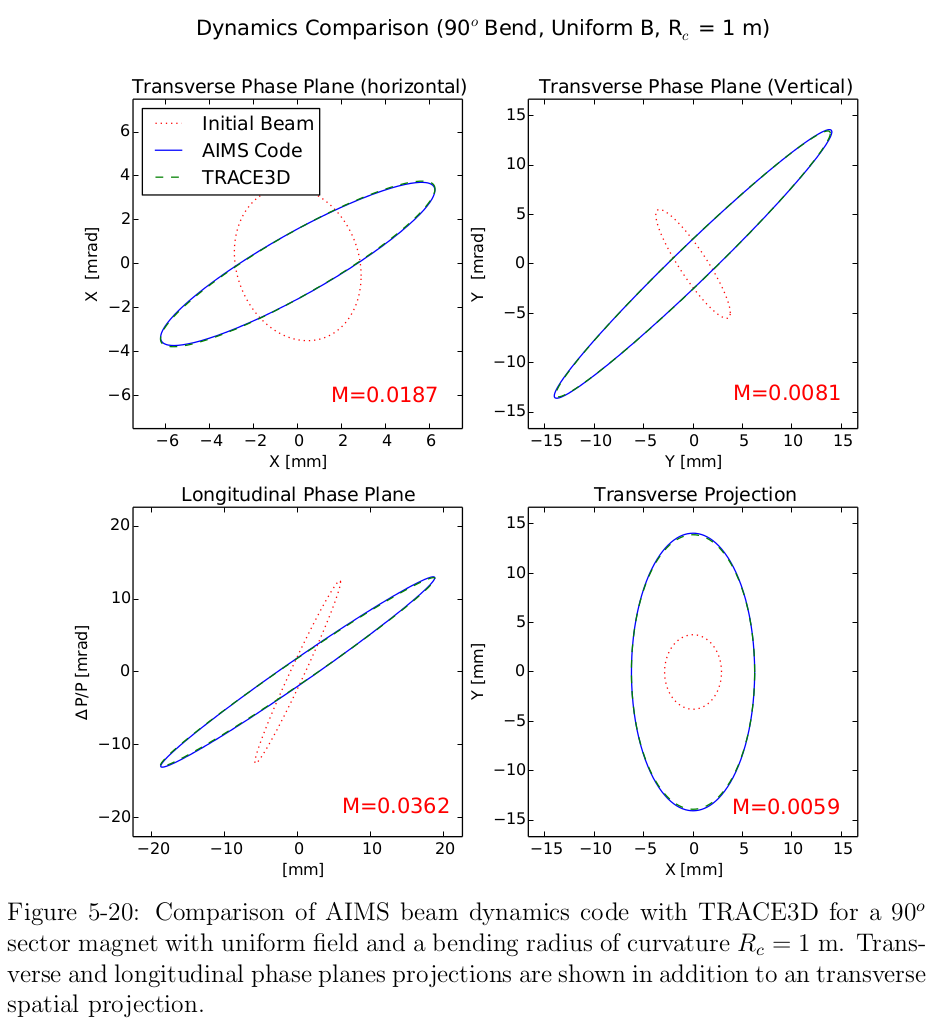CV

Projects

Research

Publications

Github

Press

# Beam Dynamics Simulations (doctoral thesis)

27 Jan 2014

####Overview:

To design and support the AIMS diagnostic developed in my doctoral thesis work, I developed a suite of ion beam dynamics simulation tools. These tools are capable of modeling classical and relativistic ion beam trajectories in the complex 3D magnetic fields. In addition, the code can model the 6D dynamics of the of the beam’s phase space distribution as it evolves due to beam curvature, magnetic field gradients, and space charge effects. In an effort generalize these simulation tools, the code has been extended to include relativistic effects making the tools applicable to high energy ion beams and electron beams.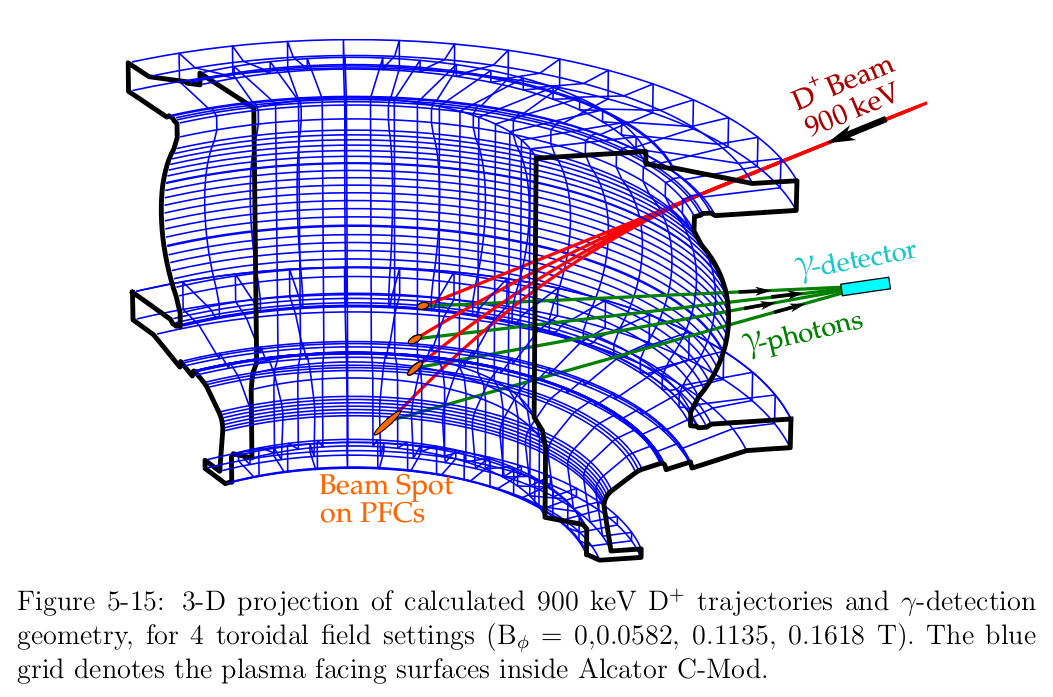The simulation tools were written in python and are available from my github repository: python-BeamDynamicsTools. These tools are functional and have been validated against Los Alamos beam transport codes (TRACE3D) for overlapping test cases but are still a work in progress. I am in the process of adding more advanced features and improving user-friendliness.

## 3D Trajectory Calculation:

A 3D simulation of particle trajectory for beam targeting is shown bellow. The trajectories can be calculated with either with the Euler integration method or the leapfrog integration method to reduce first order error.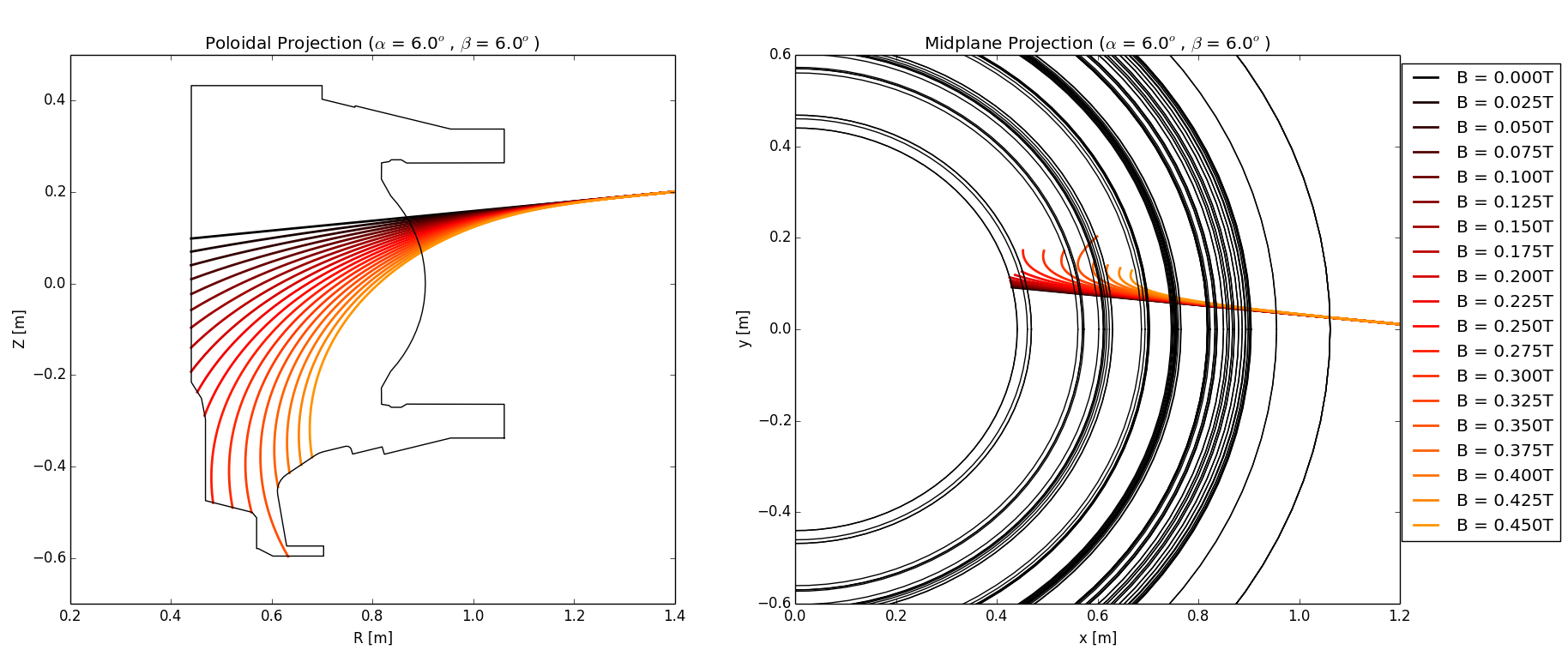While calculating the trajectory, the local position, velocity, and basis vectors along with the magnetic fields and gradients are calculated to be used later in the dynamics calculation. For accurate trajectories to be calculated, the magnetic fields must be modeled. In the case of tokamak, which is symmetric about the z-axis, the symmetry can be exploited allowing 2D field models to be used.

The toroidal field is calculated using a model that represents each toroidal field coil a vertical current filaments. The equations and field magnitude plot are shown below.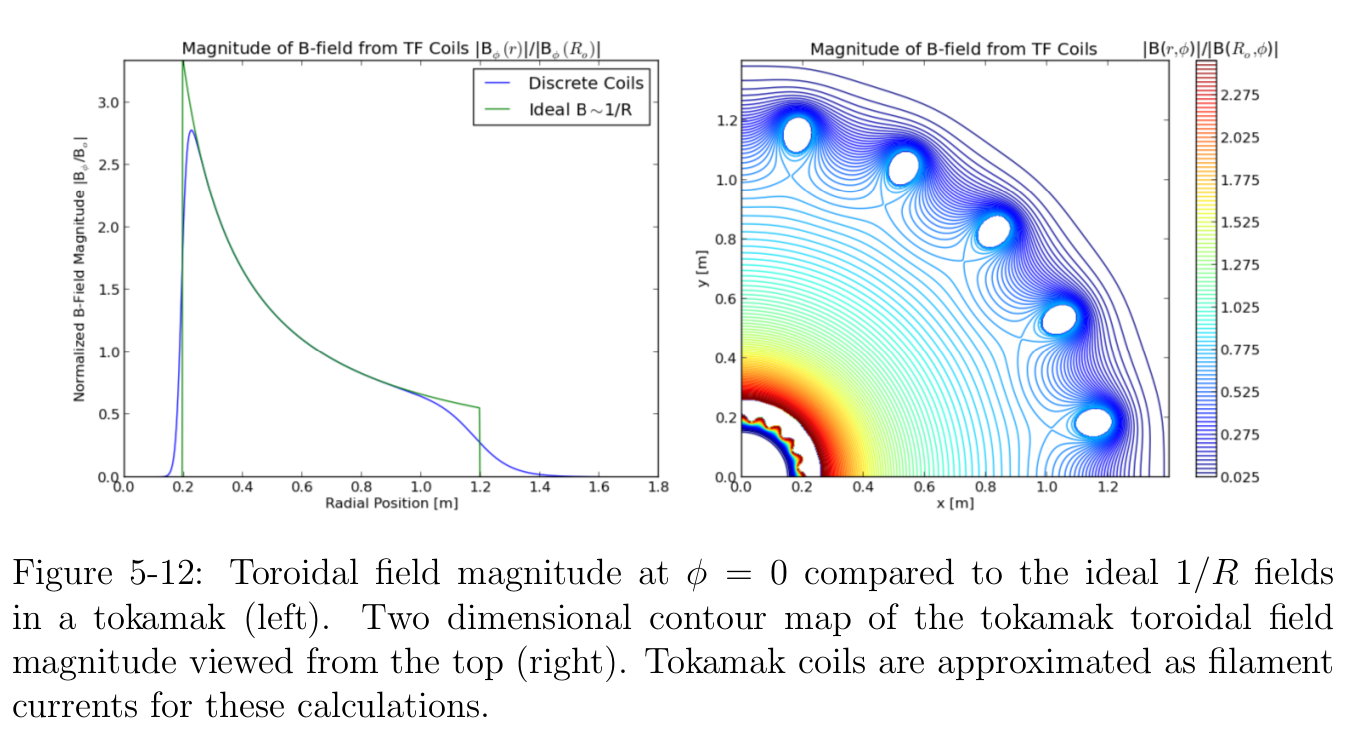The vertical field is calculated using the elliptic integral solution for off axis current loops. This accurately represents the fields create by the tokamak’s pair of vertical field coils. The equations and field plots are shown below.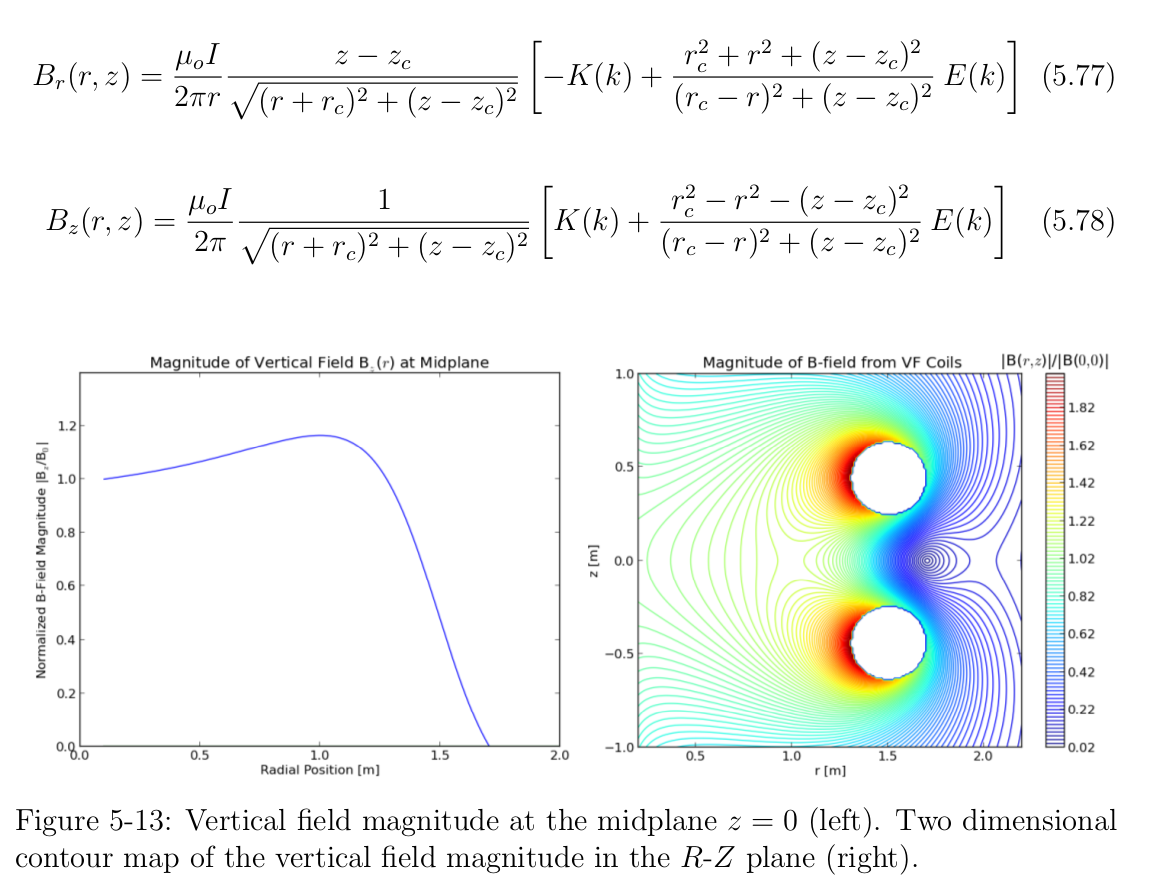From observing the beams trajectory through the magnetic fields, it is clear that the beam experiences strong field gradients and non-uniformities as it passes the coil sets in the tokamak which can cause complex focusing / defocusing effects.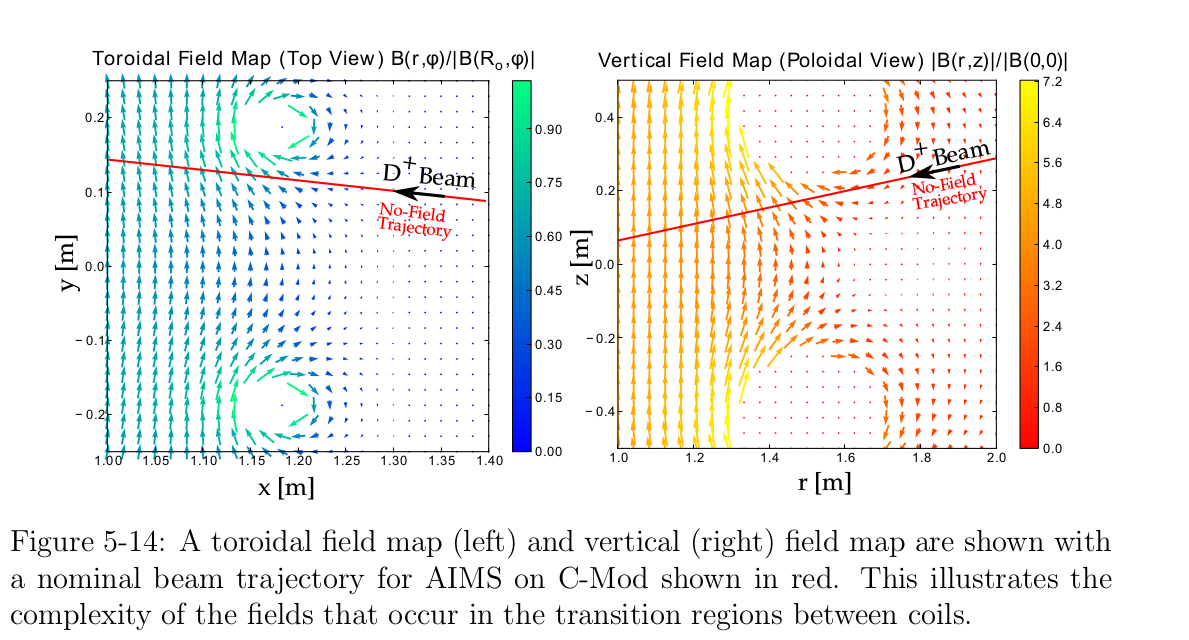The code can be further generalized by applying field maps that are calculated in using other finite element analysis packages.

## 6D Dynamics Calculation

The non-uniform fields and coupling between momentum components make it necessary to simulate the evolution of the beam’s entire distribution. For example, the field gradients and even uniform fields can effect the focusing of the beam.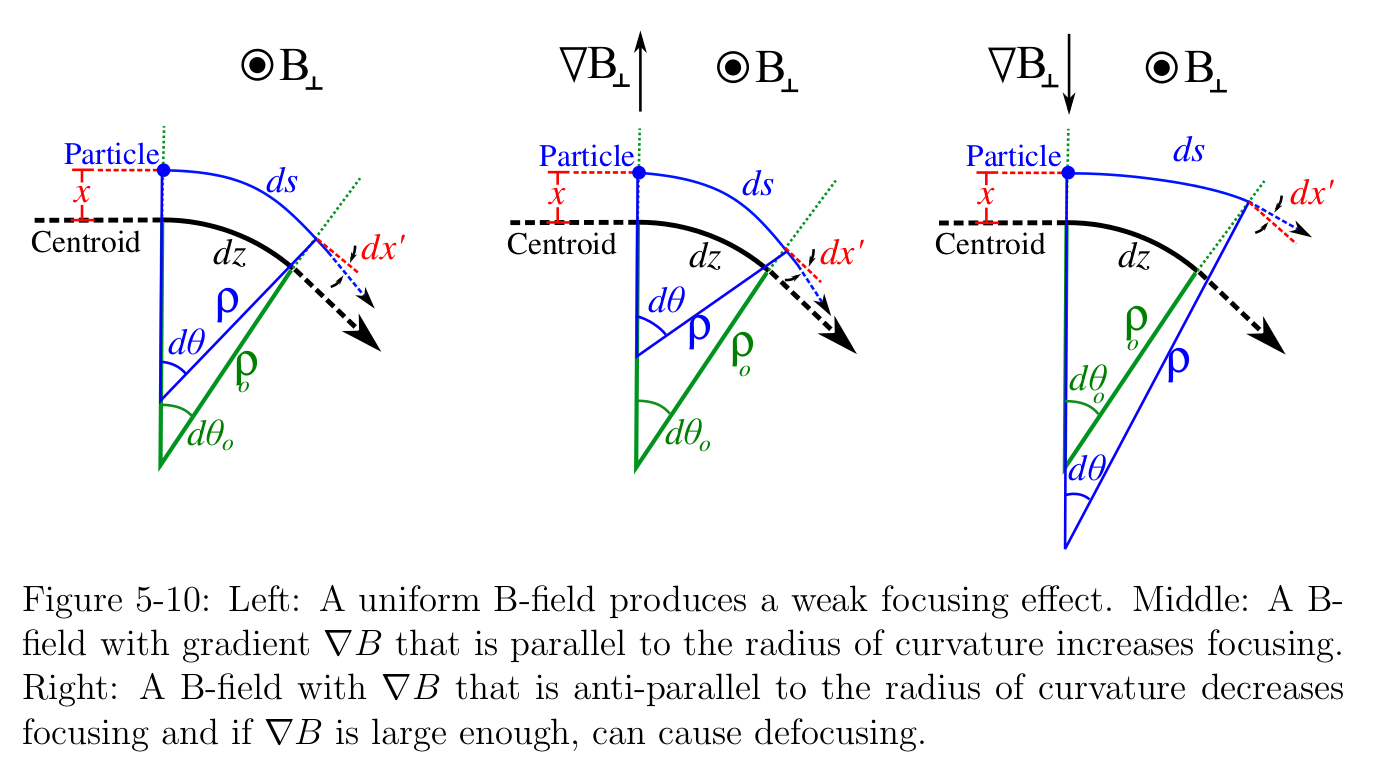In addition the spatial and momentum distribution (non-laminar) of the beam combined with fields created within the beam (space charge effects) add further complexity to the simulation.For the AIMS diagnostic, the beam currents and beam emittance fall in the region where both space charge effects and non-laminar, emittance drive effects contribute to the beam’s evolution as shown in the following plot: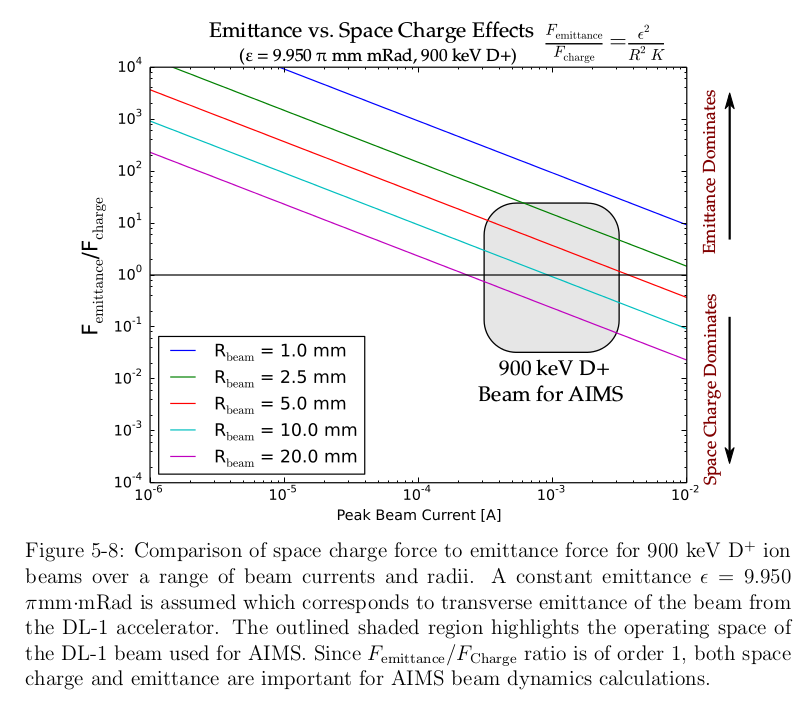The simulation must include the beam’s the 3 spatial dimensions and the beams 3 momentum dimensions. The distribution is represented by as a 6D ellipsoidal envelope enclosing the phase volume of the beam. This ellipsoidal envelope is described using the sigma matrix formalism where the ellipse parameters from each phase plane are combined into a 6x6 matrix form which is operated on by transfer matrices. Linear models are used to define these transfer matrices at each integration step along the trajectory to model the beam’s evolution as it passes through fields and due to space charge. For example, the transfer matrix for the magnetic field effects are shown below for a beam with charge Q, momentum Po, and local magnetic field B: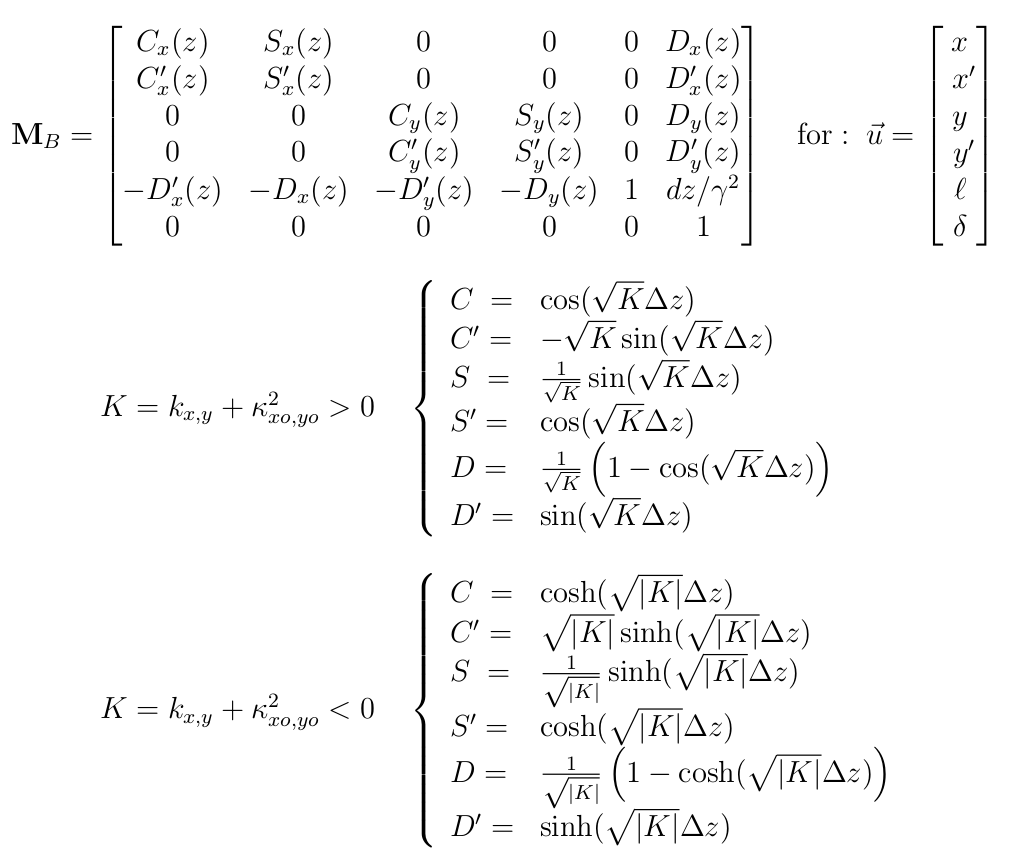Combining all of the effects, the beam simulation code has successfully been used to calculate the beam trajectories and beam target distributions for the AIMS diagnostic on the Alcator C-Mod tokamak at MIT.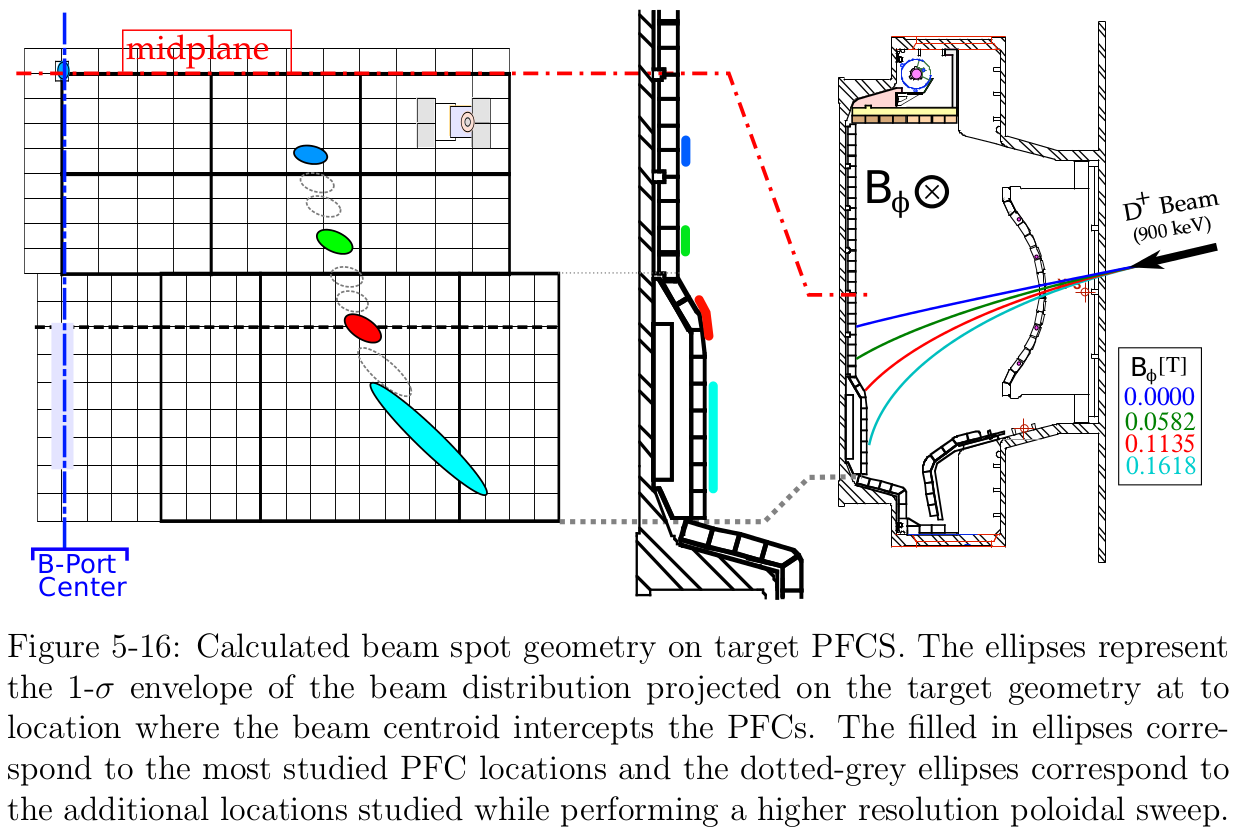## Code Validation:

Furthermore, the beam beam dynamics code has been validated against the TRACE3D beam transport code developed at Los Alamos. Phase ellipse plots for an overlapping test case (one of many) shows exact agreement with between the beam dynamics code and the TRACE3D.